Quick Notes Moles - Solutions

• The number of moles of a solute in a solution can be calculated using the formula:• Volume (of solution) is usually given in cm3 and must be converted into dm3.
• 1 dm3 is the same as 1000 cm3
• To convert dm3 to cm3 x 1000
• To convert cm3 to dm3 / 1000
• Concentration refers to how many moles of solute are in 1dm3 of solution (units are mol dm-3).

Full Notes Moles - Solutions

Remember there are two main ways of measuring how much of a substance you have – mass and volume.

When a substance is dissolved in water (a solution), mass is not very useful measurement when calculating the number of moles of the substance. Instead, we need to use the volume and concentration of the solution.

Volume is a measurement of the amount of space a substance occupies and is given in cm3 or dm3.

Concentration is just a way of describing how much of a substance we have in a given volume of solution. The more solute there is in a given volume, the more concentrated the solution is.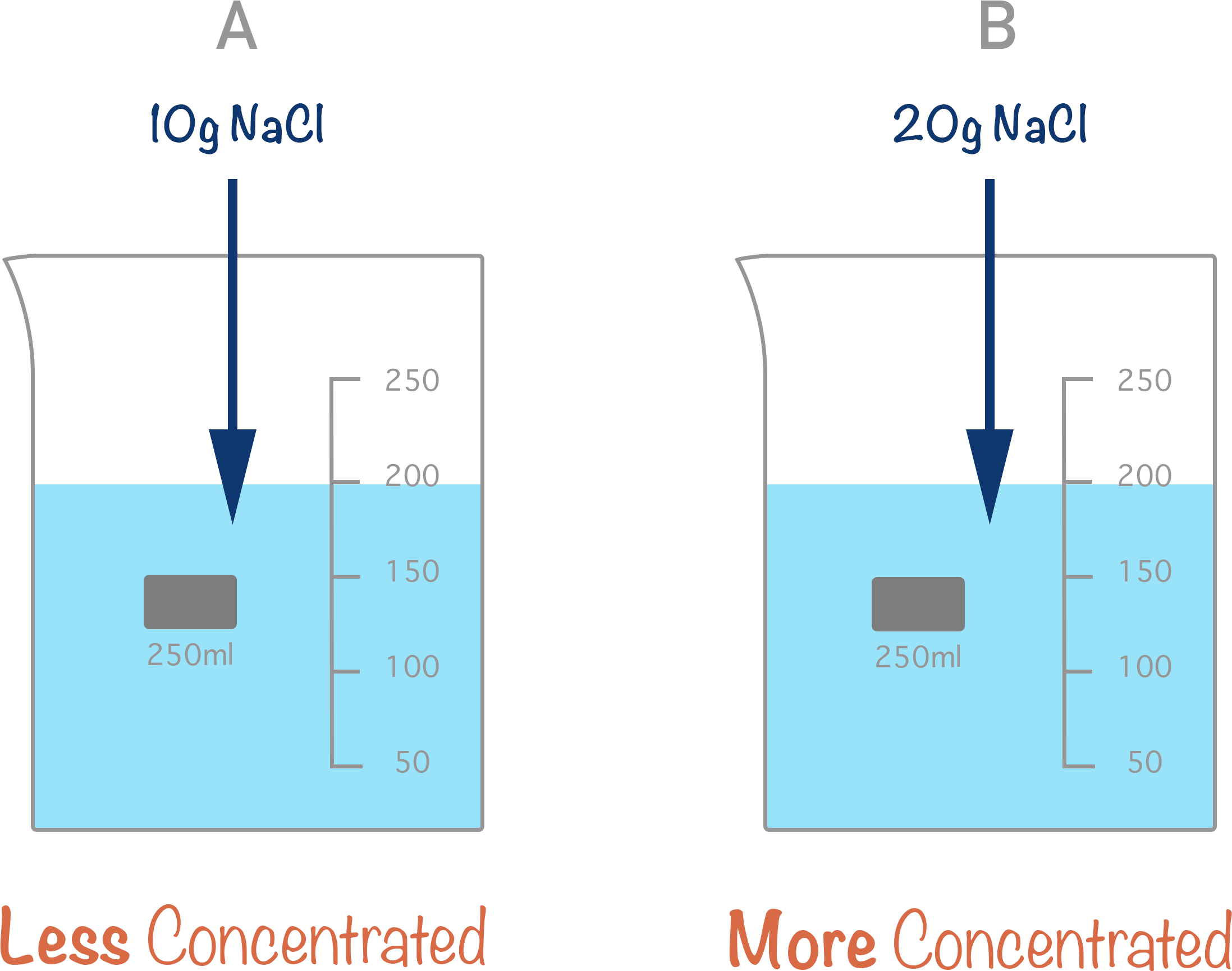In chemistry, the standard definition for concentration is moles per decimetre cubed (moles per dm3). Sometimes you will see concentration written as ‘M’ for molarity – this is the same thing when dealing with solutions. Molarity just describes how many moles of a substance we have in 1 litre of solution (1 litre = 1000 cm3).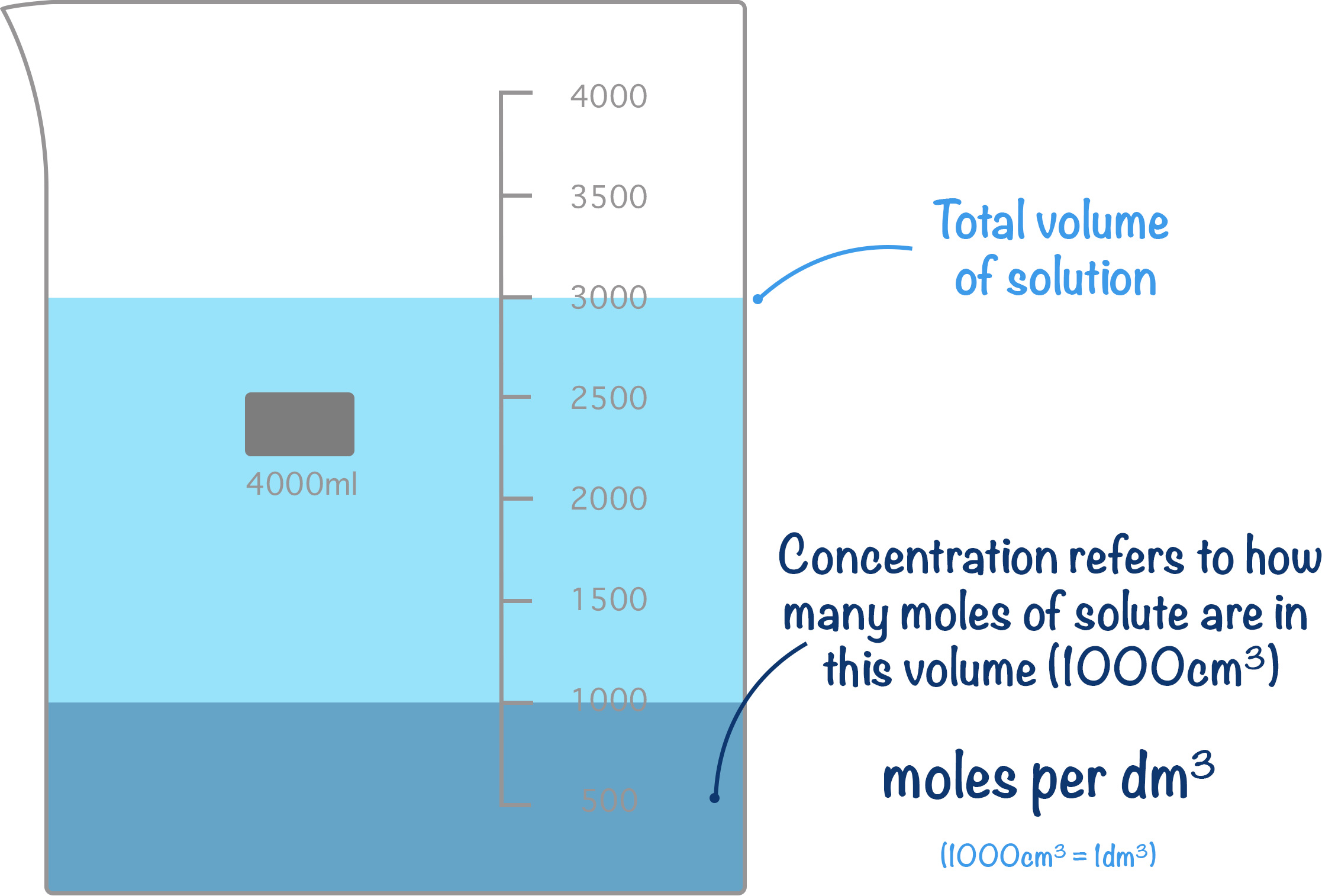If a solution of hydrochloric acid has a concentration of 2 mol dm-3, then 1 dm3 of solution would have 2 moles’ worth of hydrochloric acid (HCl) molecules in it. Equally, if a solution of sodium hydroxide has a concentration of 4 mol dm-3, then 1 dm3 of solution would have 4 moles’ worth of sodium hydroxide (NaOH). It doesn’t matter what the overall volume of solution is, the concentration is always given in moles per dm3.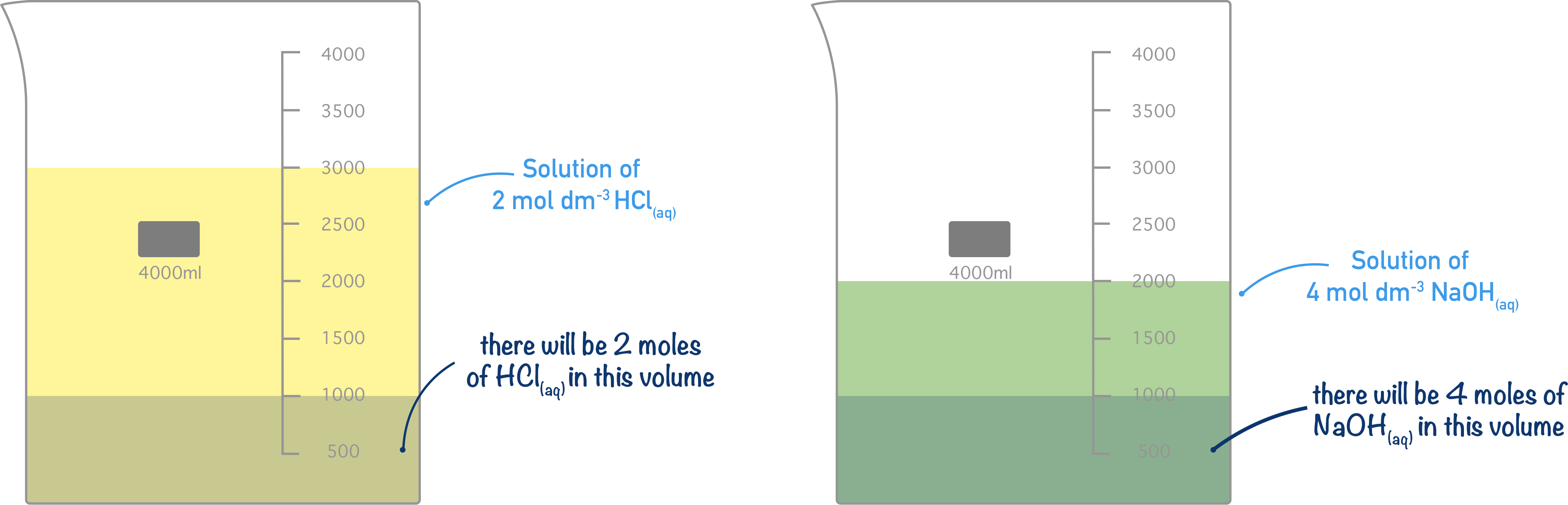If the volume and the concentration of a solution is known, it is possible to calculate how many moles of a substance there are in that volume.

The equation used is straightforward, but you need to able to use it well.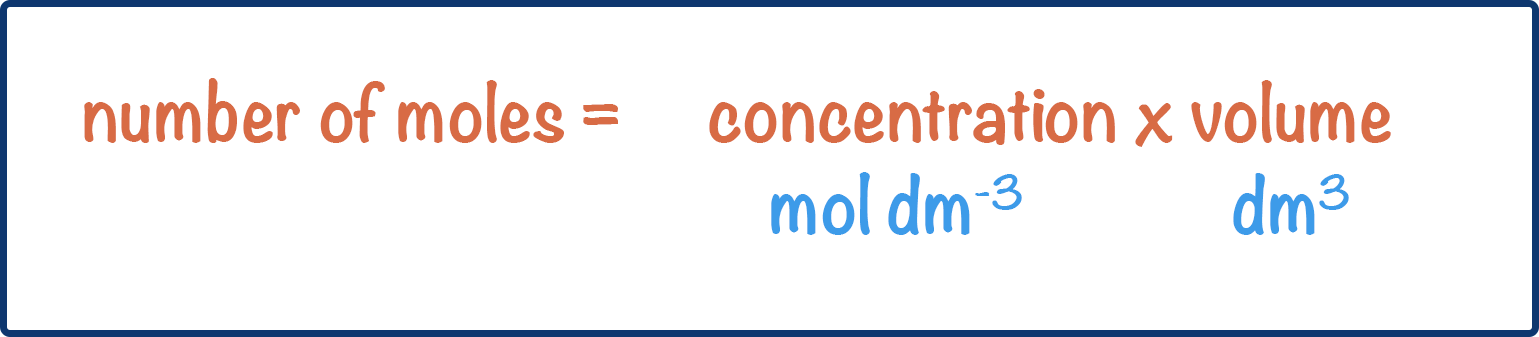number of moles = concentration (moles per dm3) x volume (dm3)

Concentration is always given as moles per dm3, but volumes can be stated as either dm3 or cm3. If a volume is given in cm3, in order to find the moles using concentration, it needs to be converted from cm3 to dm3. To do this, it is divided by 1000.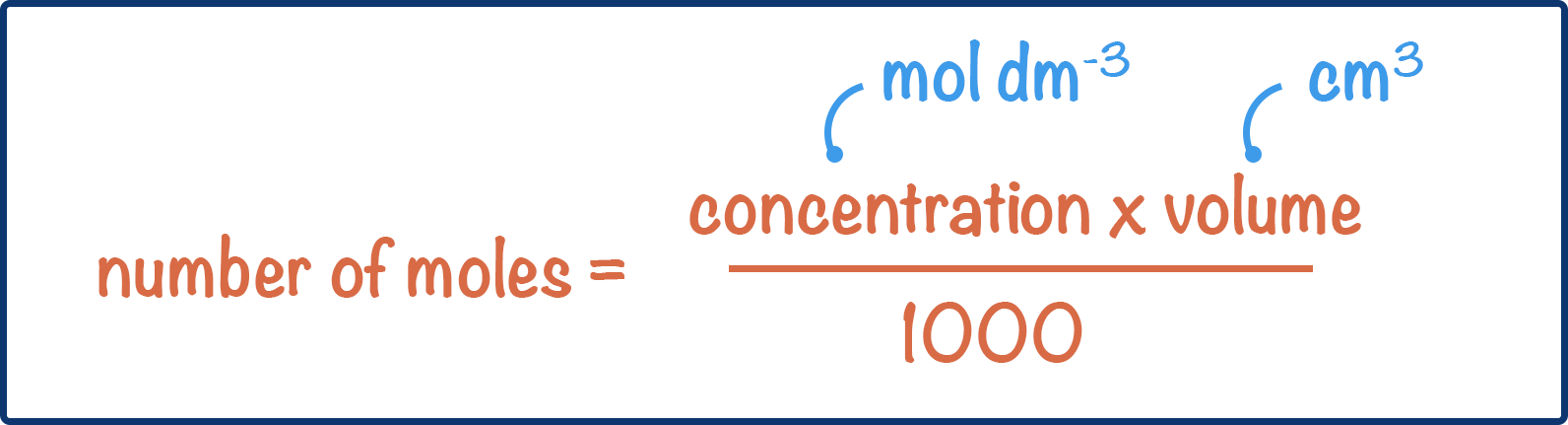number of moles = concentration (moles per dm3) x volume (cm3) / 1000